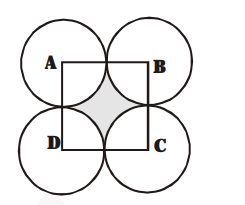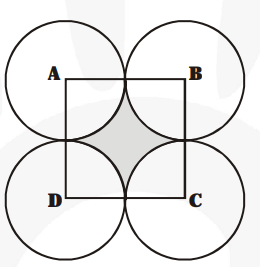# In fig., ABCD is a square of side 14 cm. With centres A, B, C and D,

Question:

In fig., ABCD is a square of side 14 cm. With centres A, B, C and D, four circles are drawn such that each circle touch externally two of the remaining three circles. Find the area of the shaded region.Solution:

Side of the square ABCD = 14 cm

$\therefore \quad$ Area of the sqaure $\mathrm{ABCD}=14 \times 14 \mathrm{~cm}^{2}$

$=196 \mathrm{~cm}^{2}$

$\because \quad$ Circles touch each other

Radius of the circle $=\frac{\mathbf{1 4}}{\mathbf{2}}=7 \mathrm{~cm}$Now, area of a sector of radius $7 \mathrm{~cm}$ and sector angle $\theta$ as $90^{\circ}$

$=\frac{90^{\circ}}{360^{\circ}} \times \frac{22}{7} \times 7 \times 7 \mathrm{~cm}^{2}=\frac{11 \times 7}{2} \mathrm{~cm}^{2}$

Area of 4 sectors

$=4 \times\left[\frac{\mathbf{1 1} \times \mathbf{7}}{\mathbf{2}}\right]=2 \times 11 \times 7 \mathrm{~cm}^{2}=154 \mathrm{~cm}^{2}$

Area of the shaded region = [Area of the square ABCD] – [Area of the 4 sectors]

$=196 \mathrm{~cm}^{2}-154 \mathrm{~cm}^{2}=42 \mathrm{~cm}^{2}$